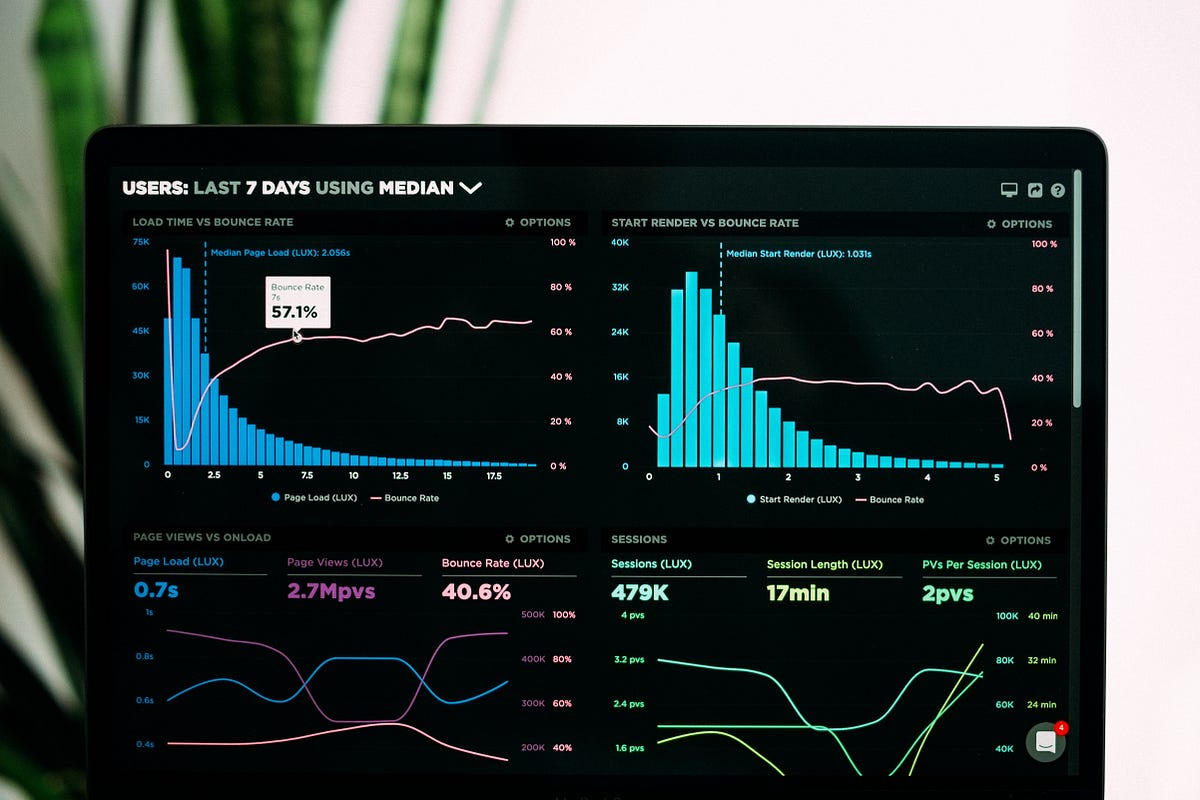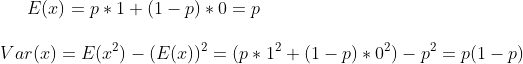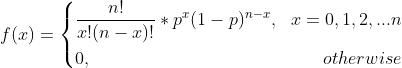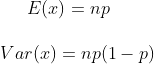# Seven Must-Know Statistical Distributions and Their Simulations for Data ScienceThis article will introduce the seven most important statistical distributions, show their Python simulations with either the Numpy library embedded functions or with a random variable generator, discuss the relationships among different distributions and their applications in data science.

A statistical distribution is a parameterized mathematical function that gives the probabilities of different outcomes for a random variable. There are discrete and continuous distributions depending on the random value it models. This article will introduce the seven most important statistical distributions, show their Python simulations with either the Numpy library embedded functions or with a random variable generator, discuss the relationships among different distributions and their applications in data science.

## Different Distributions and Simulations

### 1, Bernoulli Distribution

Bernoulli distribution is a discrete distribution. The assumptions of Bernoulli distribution include:

1, only two outcomes;

2, only one trial.

Bernoulli distribution describes a random variable that only contains two outcomes. For example, when tossing a coin one time, you can only get “Head” or “Tail.” We can also generalize it by defining the outcomes as “success” and “failure.” If I assume that when I toss a die, I only care if I get six, I can define the outcome of a die showing six as “success” and all other outcomes as “failure.” Even though tossing a die has six outcomes, in this experiment that I define, there are only two outcomes, and I can use Bernoulli distribution.

The probability mass function (PMF) of a random variable x that follows the Bernoulli distribution is:

p is the probability that this random variable x equals ‘success,’ which is defined based on different scenarios. Sometimes we have p = 1-p, like when tossing a fair coin.

From the PMF, we can calculate the expected value and variance of random variable x depending on the numerical value of x. If x=1 when “success” and x=0 when “failure,” E (x) and Var (x) are:Simulating a Bernoulli trial is straightforward by defining a random variable that only generates two outcomes with a certain “success” probability p:

``````import numpy as np

#success probability is the same as failure probability
np.random.choice([‘success’,’failure’], p=(0.5, 0.5))
#probabilities are different
np.random.choice(['success','failure'], p=(0.9, 0.1))``````

### 2, Binomial Distribution

Binomial distribution is also a discrete distribution, and it describes the random variable x as the number of success in n Bernoulli trials. You can think of the binomial distribution as the outcome distribution of n identical Bernoulli distributed random variables. The assumptions of the Binomial distribution are:

1, each trial only has two outcomes (like tossing a coin);

2, there are n identical trials in total (tossing the same coin for n times);

3, each trial is independent of other trials (getting “Head” at the first trial wouldn’t affect the chance of getting “Head” at the second trial);

4, p, and 1-p are the same for all trials (the chance of getting “Head” is the same across all trials);

There are two parameters in the distribution, the success probability p and the number of trials n. The PMF is defined using the combination formula:The probability that we have x number of success out of n trials is like choosing x out of n when order doesn’t matter.

Thinking about Binomial distribution as n identical Bernoulli distributions helps understand the calculation of its expected value and variance:If you are interested in getting these two equations above, you can watch these wonderful videos from Khan Academy.

Python’s Numpy library has a built-in Binomial distribution function. To simulate it, define n and p, and set to simulate 10000 times:

``````n = 100
p = 0.5
size = 10000

binomial = np.random.binomial(n,p,1000)
plt.hist(binomial)``````

## Exploratory Data Analysis is a significant part of Data Science

Data science is omnipresent to advanced statistical and machine learning methods. For whatever length of time that there is data to analyse, the need to investigate is obvious.

## 50 Data Science Jobs That Opened Just Last Week

Data Science and Analytics market evolves to adapt to the constantly changing economic and business environments. Our latest survey report suggests that as the overall Data Science and Analytics market evolves to adapt to the constantly changing economic and business environments, data scientists and AI practitioners should be aware of the skills and tools that the broader community is working on. A good grip in these skills will further help data science enthusiasts to get the best jobs that various industries in their data science functions are offering.

## Learn Data Science using CRISP-DM Framework

If you’re interested in the exciting world of data science, but don’t know where to start, CRISP-DM Framework is here to help.

## Basic statistics in Data science

The term “Data science” comes in 1996 which was included in the title of a statistical conference (International Federation of…

## Statistical Tests for Data Analysis Part-I

These statistical tests allow researchers to make inferences because they can show whether an observed pattern is due to intervention or chance. There is a wide range of statistical tests.